Latest Banking jobs   »

# Quantitative Aptitude Quiz For Bank of Baroda AO 2023 -30th March

Q1. Q is 450 more than P. 60% of P is 60 more than 45% of Q. Then find sum of P and Q ?
(a) 3950
(b) 3750
(c) 3850
(d) 3650
(e) 4050
Q2. Raheem spends 36% of his monthly income on daily spending, 40% on house rent and children fee together and rest amount he saves for future needs. If his total savings be Rs. 14,400. Find his total monthly income.
(a) Rs. 45,000
(b) Rs. 40,000
(c) Rs. 48,000
(d) Rs. 60,000
(e) Rs. 55,000

Q3. The population a city after three years will be 21,600. If rate of increase of population per year be 20% then find the present population of the city.
(a) 12,500
(b) 16,500
(c) 14,500
(d) 10,500
(e) 11,600
Q4. In an election between two candidates, one got 55% of total valid votes and 20% of the total votes casted were invalid. If total votes were 7500, then what is the number of valid votes that the other person got?
(a) 2550
(b) 2670
(c) 2700
(d) 2850
(e) 2500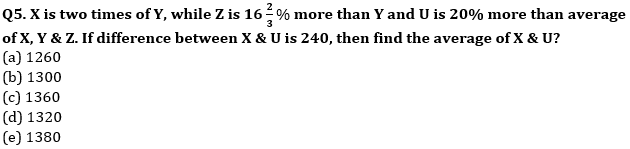Q6. The ratio of number of balls in bags x, y is 2 : 3. Five ball are taken from bag y and are dropped in bag x, number of balls are equal in each bag now. Number of balls in each bag now is
(a) 45
(b) 20
(c) 30
(d) 25
(e) 35
Q7. A and B earn in the ratio 2 : 1. They spend in the ratio 5 : 3 and save in the ratio 4 : 1. If the total monthly savings of both A and B are Rs. 5,000, the monthly income of B is
(a) Rs. 7,000
(b) Rs. 14,000
(c) Rs. 5,000
(d) Rs. 10,000
(e) Rs. 12,000
Q8. Ratio of two numbers is 5 : 7. If 30 is added in each number, the ratio becomes 3 : 4. Find the ratio of the number if 10 is subtracted from each number.
(a) 5 : 8
(b) 8 : 11
(c) 7 : 10
(d) 6 : 11
(e) 7 : 9

Q9. Two numbers A and B are in ratio 11 : 19, when 19 is added to A and 11 is added to B the ratio becomes 2 : 3, Find value of (20 + B)?
(a) 154
(b) 144
(c) 164
(d) 133
(e) 153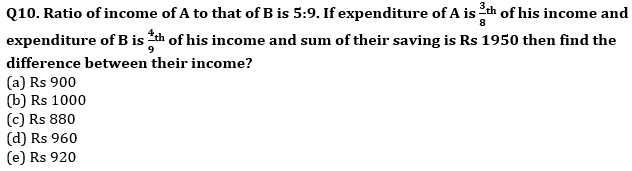Q11. The average weight of ten students of class is 40 kg. If the lightest and heaviest student are not taken into account then average weight of remaining students is 41 kg. If the weight of heaviest student is 50 kg, find the weight of lightest student. (in Kg)
(a) 21
(b) 22
(c) 23
(d) 24
(e)25
Q12. The average of ‘x’ numbers is 24. If 1/4 of the numbers are increased by 6 each and remaining are decreased by 4 each then what is the new average?
(a) 21.5
(b) 22.5
(c) can’t be determined
(d) 23
(e)none of these
Q13. Average salary of Mark & John is Rs. 25000 while that of John & Shane is Rs. 30000. If the average salary of all 3 is Rs. 27000. Mac’s salary is Rs. 4000 more than that of John. If all
the 4 members salaries are included, what will be the new average salary? (in Rs.)
(a) 27500
(b) 31000
(c) 28000
(d) 28500
(e) 29000
Q14. The average age of 25 men are 60 yrs. If another 5 men are added whose average age is 30 yrs, then find the average age of total men?
(a) 50 yrs
(b) 55 yrs
(c) 60 yrs
(d) 45 yrs
(e) 52 yrs
Q15. Average marks of Bunty, Shunty & Chunky in an exam is 90 while that of Dumpty is 20% more than average marks of Shunty. If average marks of Bunty & Chunky is 95. Find average marks of all four.
(a) 91.5
(b) 93.5
(c) 88.5
(d) 90
(e) 95

Solutions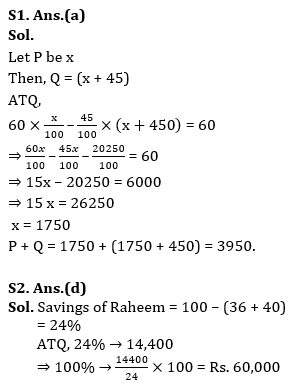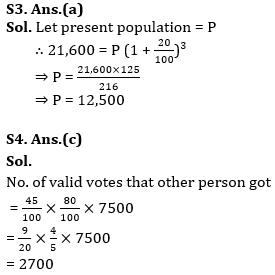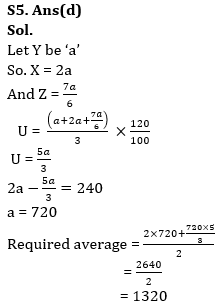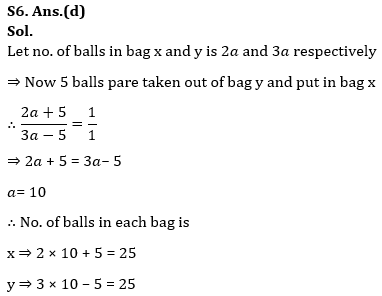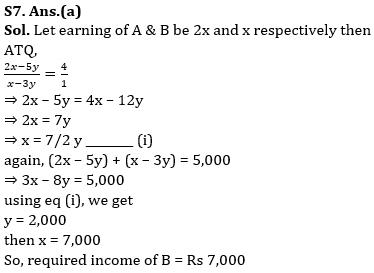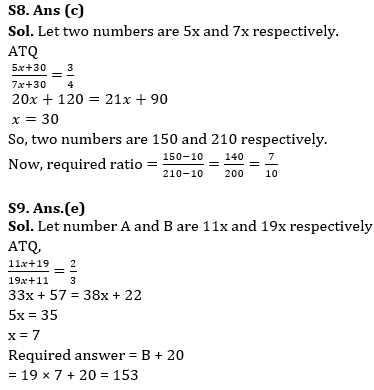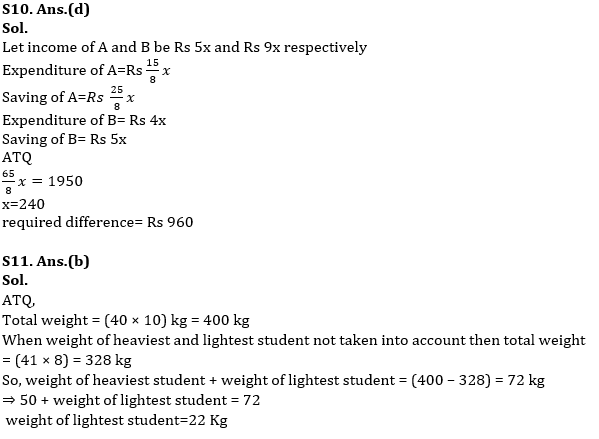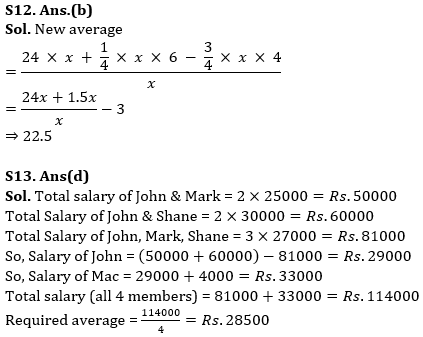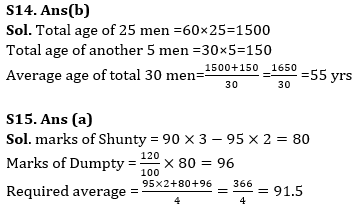.

## FAQs

### What is the selection process of Bank of Baroda AO 2023?

The selection process of Bank of Baroda AO consists of Online exam and Interview

#### Congratulations!Union Budget 2023-24: Free PDF